Home » SQL Server FLOOR Function

# SQL Server FLOOR Function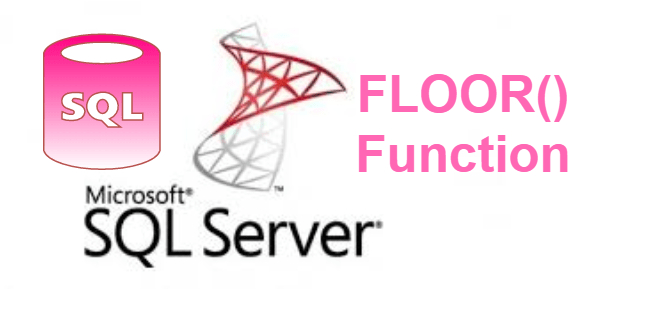### SQL Server FLOOR Function is a mathematical function that returns the largest integer value that is less than or equal to a number.

SYNTAX

`FLOOR ( numeric_expression )`

numeric_expression
Is a numeric input value or approximate numeric data type category.

Lets look at an example of FLOOR() function in SQL Server.

## FLOOR function with positive values

For input value 100.01

` SELECT FLOOR(100.01) AS OUTPUT`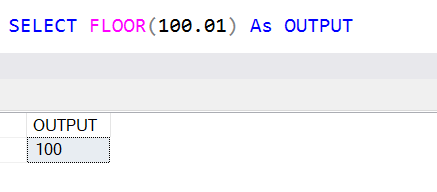It returns 100, which is a largest integer value that is less than input value 100.01.

For input value 155.61

` SELECT FLOOR(155.61) AS OUTPUT`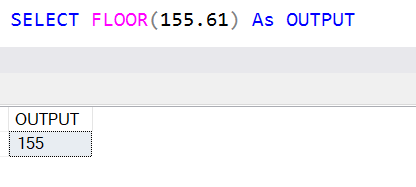It returns 155, which is a largest integer value that is less than input value 155.61.

For input value 00.50

`SELECT FLOOR(00.50) AS OUTPUT`It returns 0, which is a largest integer value that is less than input value 00.50.

For input value 568.01

`SELECT FLOOR(568.01) AS OUTPUT`It returns 568, which is a largest integer value that is less than input value 568.01.

## FLOOR function with positve values

For Negative input value -00.01

`SELECT FLOOR(-00.01) AS OUTPUT`It returns -1, which is a largest integer value that is less than input value -00.01.

For Negative input value -555.12

`SELECT FLOOR(-555.12) AS OUTPUT`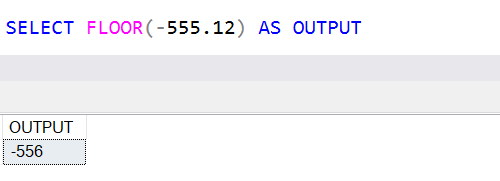It returns -556, which is a largest integer value that is less than input value -555.12.

For Negative input value -568.01

`SELECT FLOOR(-568.01) AS OUTPUT`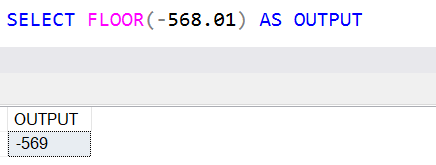It returns -569, which is a largest integer value that is less than input value -568.01.